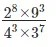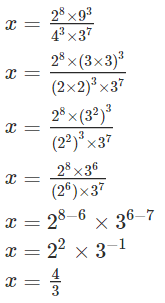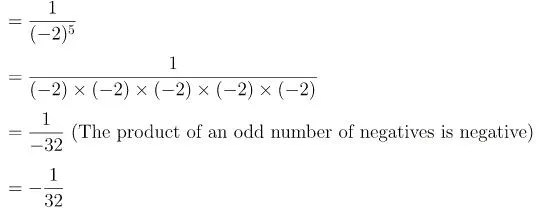# Points to Remembe of Exponents and Power

Points to Remembe of Exponents and Power

• The numbers with negative exponents also obey the following laws:
(i) xm * xn = xm+n
(ii) xm ÷ xn = xm–n
(iii) xm * bm = (xb)m
(iv) x0 = 1• A number is said to be in the standard form, if it is expressed as the product of a number between 1 and 10 and the integral power of 10.
• Very small numbers can be expressed in standard form using negative exponents.

We Know That

When we write 54, it means 5 * 5 * 5 * 5, i.e. 5 is multiplied 4 times. So 5 is the base and 4 is the exponent. We read 54 as “5 raised to the power 4’’.We also know the following laws of exponents
(i) xm ⋅ xn = xm+n
(ii) xm ÷ xn = xm–n
(iii) (xm)n = xm*n
(iv) xm * ym = (xy)m

The value of any number raised to 0 is 1, i.e. a0 = 1.
We express very small or very large numbers in standard form (i.e scientific notation) for
Example:

(ii) 3600000000000 = 36 * 1011 = 3.6 * 1012
So, 3.6 * 1012 is the standard form.

Power with Negative Exponents

For a non-zero integer x, we have

or  x–m * xm = 1
So x–m is the reciprocal (or the multiplicative inverse) of xm and vice versa.
For example: (i) Reciprocal of 8–7 = 87 and
(ii) Reciprocal of 8= 8–7

Solved Examples

Ques 1: Find the multiplicative inverse of the following.
(i) 2–4
(ii) 10–5
(iii) 7–2
(iv) 5–3
(v) 10–100
Solution:
(i) The multiplicative inverse of 2–4 is 24.
(ii) The multiplicative inverse of 10–5 is 105
(iii) The multiplicative inverse of 7–2 is 72.
(iv) The multiplicative inverse of 5–3 is 53.
(v) The multiplicative inverse of 10–100 is 10100.

Ques 2: Find the value ofSolution:
By using rule 2 and 3 –Ques 3: Solve the following: (-3)2 × (5/3)3
Solution:
(-3)2 × (5/3)3
= (-3 × -3) × ( ( 5 × 5 × 5 ) / ( 3 × 3 × 3 ) )
= 9 × (125/27)
= (125/3)

Ques 4: If x11 = y0 and x=2y, then y is equal to
a. 1/2
b. 1
c. -1
d. -2
Solution:
Option A. x11 = y0 => x11 = 1 => x = 1. Given, x = 2y hence, y = x/2 =1/2

Ques 5: By what number (4)-3 be multiplied so that the product become 1/16?
Solution:
4 Simplest way to to solve this would be:
1/16 = 1/42 = (4)-2
(4)-3 × 4 = (4)-2

Ques 6: What is the value of 63 ?
a. 18
b. 216
c. 729
d. 1296

Solution: Option b.

63
= 6 × 6 × 6
= 36 × 6
= 216

Ques 7: What is the value of (-2)-5?
a. -0.03125
b. 0.03125
c. 10
d. 32

Solution: Option A.
(-2)-5= -0.03125

Scroll to Top